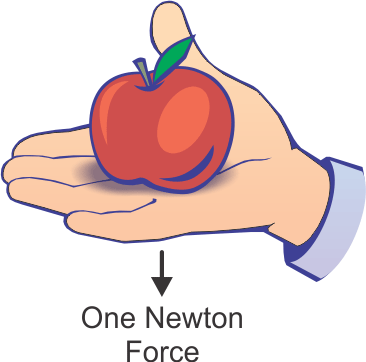# What is a Force Gauge?People are often confused about the difference between weight (mass*) and force. Scales measure weight (mass*) in pounds (lb) or kilograms (kg), etc. Force gauges, on the other hand, measure force in Newtons. (In the US, other units such as lbf, ozf and kgf are also in use.) One Newton is defined as the force needed to accelerate 1 kilogram of mass at the rate of 1 meter per second every second. You can feel this one Newton force by putting a small apple (100 g) on your palm.

The apple’s weight (mass*) is constant anywhere in the universe. When dropped, it falls freely to the Earth. In outer space, instead of falling it floats, even though its weight (mass*) is unchanged. What causes the apple to fall or float? The force caused by the object’s weight (mass*) and gravity acceleration, or as derived from Newton’s Second Law of Motion: force = mass times acceleration.

On Earth the apple falls at a continually increasing rate of speed called gravity acceleration. Gravity acceleration varies depending upon latitude, elevation and other factors. Gravity acceleration at 45 degrees latitude, sea level is 9.80665 m/S2. In 1901, the General Conference on Weights and Measures set 9.80665 m/S2 as the standard International Gravity Acceleration value.To account for the variance in gravity acceleration at different latitudes and elevations, scales need to be calibrated at and used at their location. A small apple weighed on a scale calibrated in Paris does not measure the apple’s correct weight in New York.

Force gauges, once calibrated for local gravity acceleration**, will measure the correct force values anywhere in the universe. A 100-lb weight (mass*) may produce more or less force than 100 lbf due to the variance in local gravity acceleration. A 100-lb weight (mass*) produces 100 lbf force only at the International Gravity Acceleration value of 9.80665 m/S2.

The formula for local force (lbf) produced by a 100-lb weight (mass*) is calculated as below:

Local Gravity Acceleration Value

International Gravity Acceleration Value of 9.80665

(m/S2)   x 100 (lbs)  =  Local Force (lbf)

* We are defining “weight” as “mass.” Read more about weight and mass at the NIST Guide to SI. http://physics.nist.gov/Pubs/SP811/sec08.html

** Force gauges can be calibrated by other means such as load cells without considering local gravity acceleration.

Links to local gravity acceleration calculators

http://www.wolframalpha.com/widgets/gallery/view.jsp?id=d34e8683df527e3555153d979bcda9cf
Calculates approximate gravity acceleration based on City, State

http://geodesy.noaa.gov/cgi-bin/grav_pdx.prl
Requires longitude, latitude and elevation

http://www.isobudgets.com/how-to-calculate-local-gravity/
Step by step method